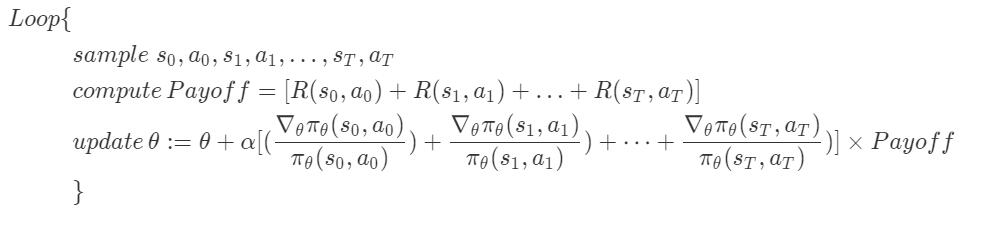# 吴恩达Stanford机器学习公开课（二十）笔记

## Lecture 20 -Policy search , Pegasus

Posted by Yunlongs on July 16, 2019

# Lecture 20 -Policy search , Pegasus

1. 定义策略集$\Pi$作为所有可能策略的合集，我们通过对集合$\Pi$进行搜索，找到可以获得最优结果的策略$π$
2. 随机策略为$π:S\times A->R$状态和动作到实数的一个映射，$\sum \pi(s, a)=1$，$π(s,a)>0$其中参数更新的推导就省略了，对目标函数使用随机梯度上升算法即可得到。 需要注意到算法中的，$[(\frac {\nabla_θ\pi_θ(s_0,a_0)}{\pi_θ(s_0,a_0)})+\frac {\nabla_θ\pi_θ(s_1,a_1)}{\pi_θ(s_1,a_1)})+ \dots +\frac {\nabla_θ\pi_θ(s_T,a_T)}{\pi_θ(s_T,a_T)})] \times Payoff = \nabla _θE[Payoff]$

## 2. Pegasus

Pegasus算法全称为Policy Evaluation of Gradient And Search Using Scenarios，使用场景进行梯度和搜索的策略评估算法。